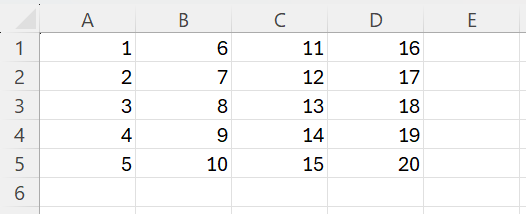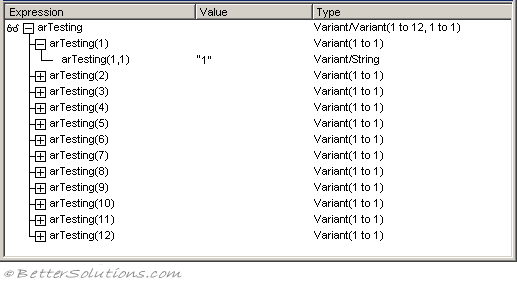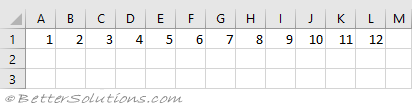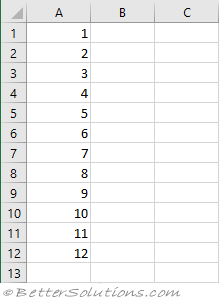# Working with Arrays

### Transfer Between Arrays with Ranges

Transferring cell values to an array and then back again after some manipulation is extremely common.
It can be more efficient to load the values from a range into an array, process the data and then write it back rather than access each cell individually.

`Dim arMyArray() As Variant arMyArray = Range("A1:D5").Value Range("A1:D5").Value = arMyArray `### Populating an Array

The quickest way to populate an array with values in a cell range is to use a simple Variant datatype
You do not need to define the size of the array before it is populated.
This is only possible when the variable is defined as a Variant.

`'both these declarations will workDim arTesting As Variant Dim arTesting() As Variant arTesting = Range("A1:A12").Value `

This will create the following type of array:
Note that this array is 2 dimensional and that it starts at 1 and not 0.
This array is always 2 dimensional even if you are referring to a single row or column.
This array always has the columns first and then the rows (check !!) with a SS### Populating a Range

The quickest way to populate a range with the contents of an array is to define the Value equal to the array.
Note that this will create a horizontal array that will populate a row across the worksheet.

`Dim arTesting As Variant arTesting = VBA.Array(1, 2, 3, 4, 5, 6, 7, 8, 9, 10, 11, 12) Range("A1:L1").Value = arTesting `

This will create a table across the worksheet.If you want to create a vertical array that will populate a column down the worksheet then you must transpose the array before assigning it to the Range.

`Dim arTesting As Variant arTesting = VBA.Array(1, 2, 3, 4, 5, 6, 7, 8, 9, 10, 11, 12) arTesting = Application.WorksheetFunction.Transpose(arTesting) Range("A1:A12").Value = arTesting `

This will create a table down the worksheet.### Empty Value

`Dim aMyArray As Variant aMyArray = Range("A1").Value `

aMyArray has a value Empty if cell "A1" is empty

`aMyArray = Range("A1").Value `

aMyArray has the value if cell "A1" contains a value

### Row and Column Vectors

`Dim aMyArray As Variant aMyArray = Range("A1:B1")    'row vector  aMyArray(1,1) = 1 aMyArray(1,2) = 2 `

`Dim aMyArray As Variant aMyArray = Range("A1:A2")    'column vector  aMyArray(1,1) = 1 aMyArray(2,1) = 2 `

### Transposing the Array

VBA > Arrays > Transposing

### Important

Excel reads FROM ranges a lot faster than it writes TO ranges.